• 链式基数排序算法
2021-03-03 11:06:19

所谓链式基数排序，就是实现基数排序时，为减少所需的辅助存储空间，应采用链表作存储结构，即链式基数排序。而基数排序也叫做多关键字排序，基数排序是一种借助“多关键字排序”的思想来实现“单关键字排序”的内部排序算法。

链式的基数排序算法解法思路(默认从小到大)： 1、以静态链表存储待排记录，并令表头指针指向第一个记录； 2、“分配” 时，按当前“关键字位”所取值，将记录分配到不同的 “链队列” 中，每个队列中记录的 “关键字位” 相同； 3、“收集”时，按当前关键字位取值从小到大将各队列首尾相链成一个链表； 4、对每个关键字位均重复 2 和 3 两步。 例：链式基数排序，下面以静态链表存储待排记录，并令表头指针指向第一个记录。  “分配” 时，按当前“关键字位”所取值，将记录分配到不同的“链队列”中，每个队列中记录的 “关键字位” 相同。 因为是 LSD，故从地位开始 ，也就是kd-1位开始，进行一趟分配：  这里的e[i]表示按哪个位进行排序变成f[i]。分别为这个队列的队头和队尾，先入先出的“链队列”或者是一个个的“桶”。 然后xx9，xx3，xx0  又遇到了 xx9，那么按照链式队列的存储方式，先进先出的入队(类似一个桶，数据从上面进入，从下面露出)  第一趟收集：按当前关键字位取值从小到大将各队列首尾相链成一个链表；(从队列的下面出去，先进先出)  进行第二趟分配：  进行第二题收集  进行第三趟分配：  进行第三趟收集  这样的话序列按照多关键字从小到大的排序有序了。 具体代码如下：

#include

#include

using namespace std;

//链式队列的节点结构，模拟桶

struct Node

{

int data;//数据域

Node *next;//指针域

};

//定义程序所需的特殊队列

class Queue

{

private:

Node *front;//链式对列的头指针

Node *rear;//链队的尾指针

public:

//构造函数，初始化队列(带头结点的链式队列)

Queue()

{

//开始先构造一个空结点，没有数据元素存储

Node *p = new Node;

p->data = NULL;

p->next = NULL;

//开始是空链队，首尾指针分别去指向队头结点

front = p;

rear = p;

}

//析构函数，销毁链队的结点占据的内存

~Queue()

{

//标记指针

Node *p = front;

//辅助的标记指针，作用是删除结点

Node *q;

//循环遍历整个队列，直到标记指针 p 为 null

while (p != NULL)

{

//比较常见的删除结点内存的写法

q = p;

//指向队列的下一个结点

p = p->next;

//销毁之

delete q;

}

}

//入队方法，从尾进入，节点不存在，需要自行创建结点的方法

void push(int e)

{

Node *p = new Node;

p->data = e;

//本结点作为了队列的尾结点

p->next = NULL;

//然后连接结点到队尾

rear->next = p;

//最后尾指针指向新的末位结点

rear = p;

}

//入队方法，尾进入，节点原来就存在的方法，不需要再新建结点和存储结点的内容

void push(Node *p)

{

//设置此结点为尾结点

p->next = NULL;

//链接结点

rear->next = p;

//尾指针指向新的尾结点

rear = p;

}

//求数据元素的最大位数的方法，也就是求出需要分配和收集的次数

int lengthData()

{

int length = 0;//保存数据元素的 最大位数

int n = 0;   //单个数据元素具有的位数

int d;      //用来存储待比较的数据元素

//指示指针

Node *p = front->next;

//遍历

while (p != NULL)

{

//取出结点的数据，也就是代比较的数据元素

d = p->data;

//如果 d 为正数，很重要的一个技巧，必须是 d 大于 0 的判断

while (d > 0)

{

//数据位数分离算法

d /= 10;

//单个数据元素的位数存储在此

n++;

}

//沿着链队后移一个元素

p = p->next;

//找出数据元素的最大位数

if (length < n)

{

length = n;

}

//重新循环往复，n 设置为0

n = 0;

}

//返回最终位数

return length;

}

//判断队列是否为空

bool empty()

{

//队头指针和队尾指针重合，说明空

if (front == rear)

{

return true;

}

//否则为不空

return false;

}

//清除队列中的元素

void clear()

{

//直接把头结点之后的链接断开

front->next = NULL;

//设置尾指针指向头结点即可，回到了构造函数初始化的情景

rear = front;

}

//输出队列中的元素,传入引用参数比较好

void print(Queue &que)

{

//第一个结点是头结点，next 才是第一个存储元素的结点

Node *p = que.front->next;

//直到尾结点为止

while (p != NULL)

{

cout << p->data << " ";

//遍历所有结点

p = p->next;

}

}

//基数排序过程

{

//声明一个指针数组，该指针数组中存放十个指针，这十个指针需要分别指向十个队列，这是模拟10个桶，因为是0-9的数字，取值范围为10

Queue *arr;

//初始化这十个队列

for (int i = 0; i < 10; i++)

{

//初始化建立头结点

arr[i] = new Queue;

}

//取得待排序数据元素中的最大位数

int maxLen = que.lengthData();

//因为是 LSD 方式，从后到前，开始比较关键字，然后分配再收集，故开始设置数据分离算法中的除数为 1

int d = 1;

//将初始队列中的元素分配到十个队列中,maxlen 代表了需要分配和收集的次数

for (int i = 0; i < maxLen; i++)

{

Node *p = que.front->next;

//辅助指针 q

Node *q;

//余数为k，则存储在arr[k]指向的链式队列(桶)中

int k;

//遍历原始序列

while (p != NULL)

{

//重要的技巧，数据分离算法过程,最后勿忘模10，取余数，分离出需要的关键字位

k = (p->data / d) % 10;

q = p->next;

//把本结点 p 加入对应的队列中

arr[k]->push(p);

//指针后移，指向下一个结点

p = q;

}

//清空原始队列

que.clear();

//分配完毕，马上将十个队列中的数据收集到原始队列中

for (int i = 0; i < 10; i++)

{

if (!arr[i]->empty())

{

//从首节点开始遍历，不是头结点开始

Node *p = arr[i]->front->next;

//辅助指针 q

Node *q;

while (p != NULL)

{

q = p->next;

//收集到原始队列中，这就是为什么每次分配完毕，需要清除原始队列

que.push(p);

p = q;

}

}

}

//一趟的分配收集完毕，最后要清空十个队列

for (int i = 0; i < 10; i++)

{

arr[i]->clear();

}

//进行下一趟的分配和收集

d *= 10;

}

//输出队列中排好序的元素

print(que);

}

};

int main(void)

{

Queue oldque;

int i;

cout << "输入 int 类型的待排序的整数序列：输入 ctrl+z 结束输入，再回车即可" << endl;

//顺序输入元素

while (cin >> i)

{

oldque.push(i);

}

//基数排序

return 0;

}

程序运行结果：  原文链接: https://www.cnblogs.com/kubixuesheng/p/4374225.html . (ps:这是我的第一篇博客，感谢那些博客大佬的资源，让我学到了好多东西，以后会对自己学到的不管是重要的知识，还是算法题解等都会在个人博客上整理，以方便自己复习和提供他人查阅。)

更多相关内容
• 1.需求分析 ①.问题描述 给出一组数据,按照最低位优先的方法完成基数排序。多关键码排序按照从最主位关键码到最次位或从最次位到最主位关键码的顺序逐次排序。链式基数排序算法
• 所谓链式基数排序，就是实现基数排序时，为减少所需的辅助存储空间，应采用链表作存储结构，即链式基数排序。而基数排序也叫做多关键字排序，基数排序是一种借助“多关键字排序”的思想来实现“单关键字排序”的内部...

所谓链式基数排序，就是实现基数排序时，为减少所需的辅助存储空间，应采用链表作存储结构，即链式基数排序。而基数排序也叫做多关键字排序，基数排序是一种借助“多关键字排序”的思想来实现“单关键字排序”的内部排序算法。

链式的基数排序算法解法思路（默认从小到大）：
1、以静态链表存储待排记录，并令表头指针指向第一个记录；
2、“分配” 时，按当前“关键字位”所取值，将记录分配到不同的 “链队列” 中，每个队列中记录的 “关键字位” 相同；
3、“收集”时，按当前关键字位取值从小到大将各队列首尾相链成一个链表；
4、对每个关键字位均重复 2 和 3 两步。
例：链式基数排序，下面以静态链表存储待排记录，并令表头指针指向第一个记录。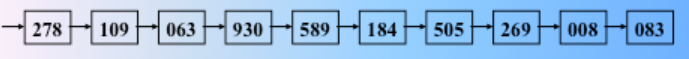“分配” 时，按当前“关键字位”所取值，将记录分配到不同的“链队列”中，每个队列中记录的 “关键字位” 相同。 因为是 LSD，故从地位开始 ，也就是kd-1位开始，进行一趟分配：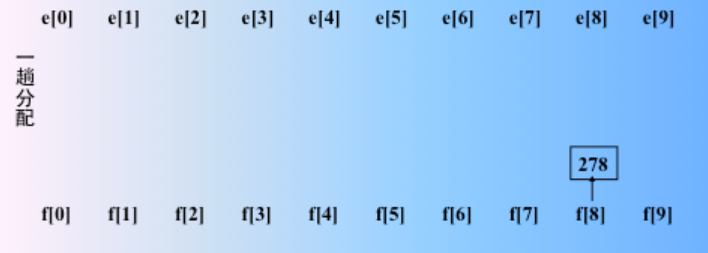这里的e[i]表示按哪个位进行排序变成f[i]。分别为这个队列的队头和队尾，先入先出的“链队列”或者是一个个的“桶”。
然后xx9，xx3，xx0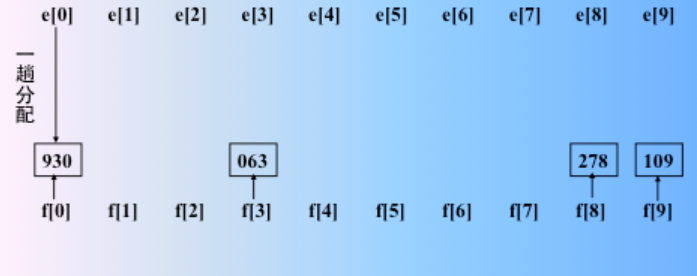又遇到了 xx9，那么按照链式队列的存储方式，先进先出的入队（类似一个桶，数据从上面进入，从下面露出）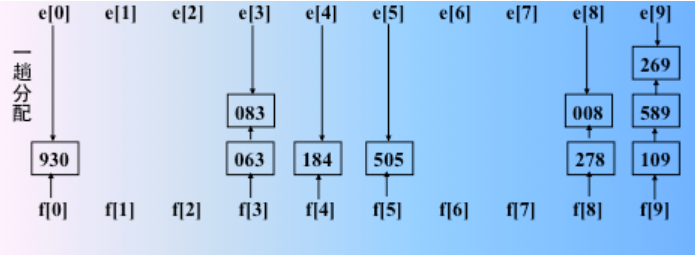第一趟收集：按当前关键字位取值从小到大将各队列首尾相链成一个链表；（从队列的下面出去，先进先出）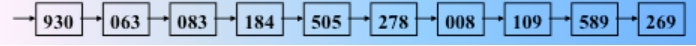进行第二趟分配：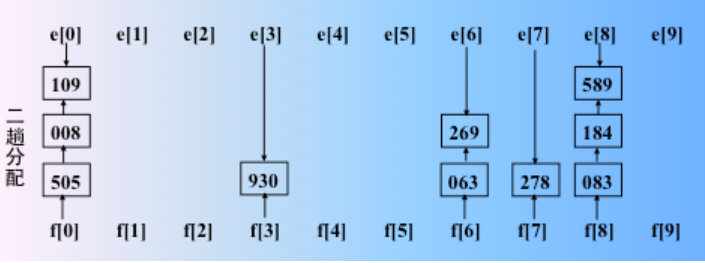进行第二题收集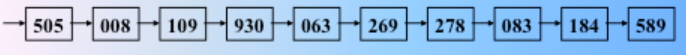进行第三趟分配：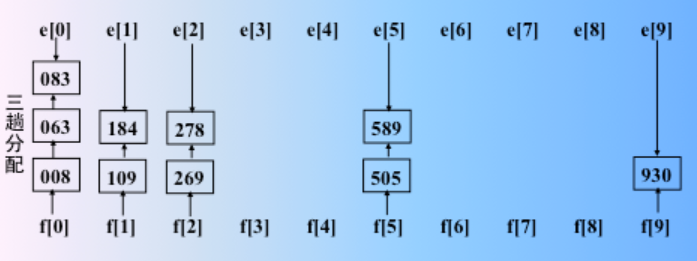进行第三趟收集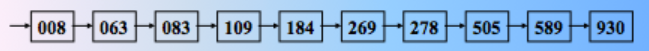这样的话序列按照多关键字从小到大的排序有序了。
具体代码如下：

#include<iostream>
#include<malloc.h>
using namespace std;
//链式队列的节点结构，模拟桶
struct Node
{
int data;//数据域
Node *next;//指针域
};
//定义程序所需的特殊队列
class Queue
{
private:
Node *front;//链式对列的头指针
Node *rear;//链队的尾指针
public:
//构造函数，初始化队列（带头结点的链式队列）
Queue()
{
//开始先构造一个空结点，没有数据元素存储
Node *p = new Node;
p->data = NULL;
p->next = NULL;
//开始是空链队，首尾指针分别去指向队头结点
front = p;
rear = p;
}
//析构函数，销毁链队的结点占据的内存
~Queue()
{
//标记指针
Node *p = front;
//辅助的标记指针，作用是删除结点
Node *q;
//循环遍历整个队列，直到标记指针 p 为 null
while (p != NULL)
{
//比较常见的删除结点内存的写法
q = p;
//指向队列的下一个结点
p = p->next;
//销毁之
delete q;
}
}
//入队方法，从尾进入，节点不存在，需要自行创建结点的方法
void push(int e)
{
Node *p = new Node;
p->data = e;
//本结点作为了队列的尾结点
p->next = NULL;
//然后连接结点到队尾
rear->next = p;
//最后尾指针指向新的末位结点
rear = p;
}
//入队方法，尾进入，节点原来就存在的方法，不需要再新建结点和存储结点的内容
void push(Node *p)
{
//设置此结点为尾结点
p->next = NULL;
//链接结点
rear->next = p;
//尾指针指向新的尾结点
rear = p;
}
//求数据元素的最大位数的方法，也就是求出需要分配和收集的次数
int lengthData()
{
int length = 0;//保存数据元素的 最大位数
int n = 0;   //单个数据元素具有的位数
int d;      //用来存储待比较的数据元素
//指示指针
Node *p = front->next;
//遍历
while (p != NULL)
{
//取出结点的数据，也就是代比较的数据元素
d = p->data;
//如果 d 为正数，很重要的一个技巧，必须是 d 大于 0 的判断
while (d > 0)
{
//数据位数分离算法
d /= 10;
//单个数据元素的位数存储在此
n++;
}
//沿着链队后移一个元素
p = p->next;
//找出数据元素的最大位数
if (length < n)
{
length = n;
}
//重新循环往复，n 设置为0
n = 0;
}
//返回最终位数
return length;
}
//判断队列是否为空
bool empty()
{
//队头指针和队尾指针重合，说明空
if (front == rear)
{
return true;
}
//否则为不空
return false;
}
//清除队列中的元素
void clear()
{
//直接把头结点之后的链接断开
front->next = NULL;
//设置尾指针指向头结点即可，回到了构造函数初始化的情景
rear = front;
}
//输出队列中的元素,传入引用参数比较好
void print(Queue &que)
{
//第一个结点是头结点，next 才是第一个存储元素的结点
Node *p = que.front->next;
//直到尾结点为止
while (p != NULL)
{
cout << p->data << " ";
//遍历所有结点
p = p->next;
}
}
//基数排序过程
{
//声明一个指针数组，该指针数组中存放十个指针，这十个指针需要分别指向十个队列，这是模拟10个桶，因为是0-9的数字，取值范围为10
Queue *arr;
//初始化这十个队列
for (int i = 0; i < 10; i++)
{
//初始化建立头结点
arr[i] = new Queue;
}
//取得待排序数据元素中的最大位数
int maxLen = que.lengthData();
//因为是 LSD 方式，从后到前，开始比较关键字，然后分配再收集，故开始设置数据分离算法中的除数为 1
int d = 1;
//将初始队列中的元素分配到十个队列中,maxlen 代表了需要分配和收集的次数
for (int i = 0; i < maxLen; i++)
{
Node *p = que.front->next;
//辅助指针 q
Node *q;
//余数为k，则存储在arr[k]指向的链式队列（桶）中
int k;
//遍历原始序列
while (p != NULL)
{
//重要的技巧，数据分离算法过程,最后勿忘模10，取余数，分离出需要的关键字位
k = (p->data / d) % 10;
q = p->next;
//把本结点 p 加入对应的队列中
arr[k]->push(p);
//指针后移，指向下一个结点
p = q;
}
//清空原始队列
que.clear();
//分配完毕，马上将十个队列中的数据收集到原始队列中
for (int i = 0; i < 10; i++)
{
if (!arr[i]->empty())
{
//从首节点开始遍历，不是头结点开始
Node *p = arr[i]->front->next;
//辅助指针 q
Node *q;
while (p != NULL)
{
q = p->next;
//收集到原始队列中，这就是为什么每次分配完毕，需要清除原始队列
que.push(p);
p = q;
}
}
}
//一趟的分配收集完毕，最后要清空十个队列
for (int i = 0; i < 10; i++)
{
arr[i]->clear();
}
//进行下一趟的分配和收集
d *= 10;
}
//输出队列中排好序的元素
print(que);
}
};

int main(void)
{
Queue oldque;
int i;

cout << "输入 int 类型的待排序的整数序列：输入 ctrl+z 结束输入，再回车即可" << endl;
//顺序输入元素
while (cin >> i)
{
oldque.push(i);
}
//基数排序

return 0;
}



程序运行结果：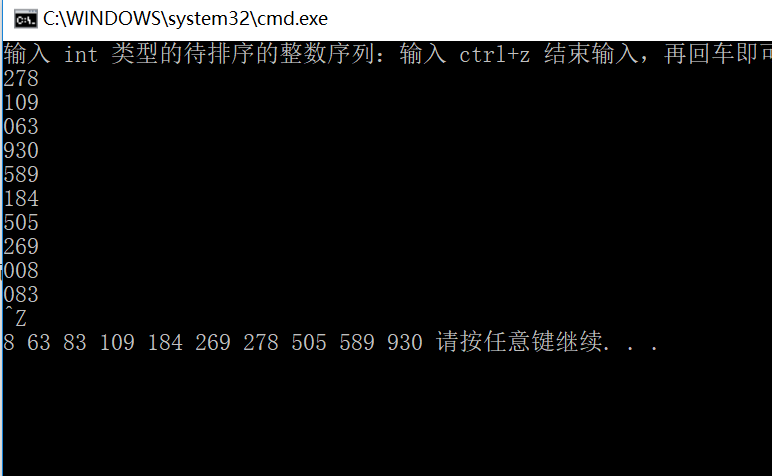原文链接: https://www.cnblogs.com/kubixuesheng/p/4374225.html

展开全文• 分配类排序不需要比较关键字的大小，它是根据关键字中各位的值，通过对待排序记录进行若干...基数排序(Radix Sorting)是典型的分配类排序。 *以上内容来自《数据结构（C语言版）（第2版）》人民邮电出版社 ...

分配类排序不需要比较关键字的大小，它是根据关键字中各位的值，通过对待排序记录进行若干趟 “ 分配 ” 与 “ 收集” 来实现排序的，是一种借助于多关键字排序的思想对单关键字排序的方法。基数排序(Radix Sorting)是典型的分配类排序。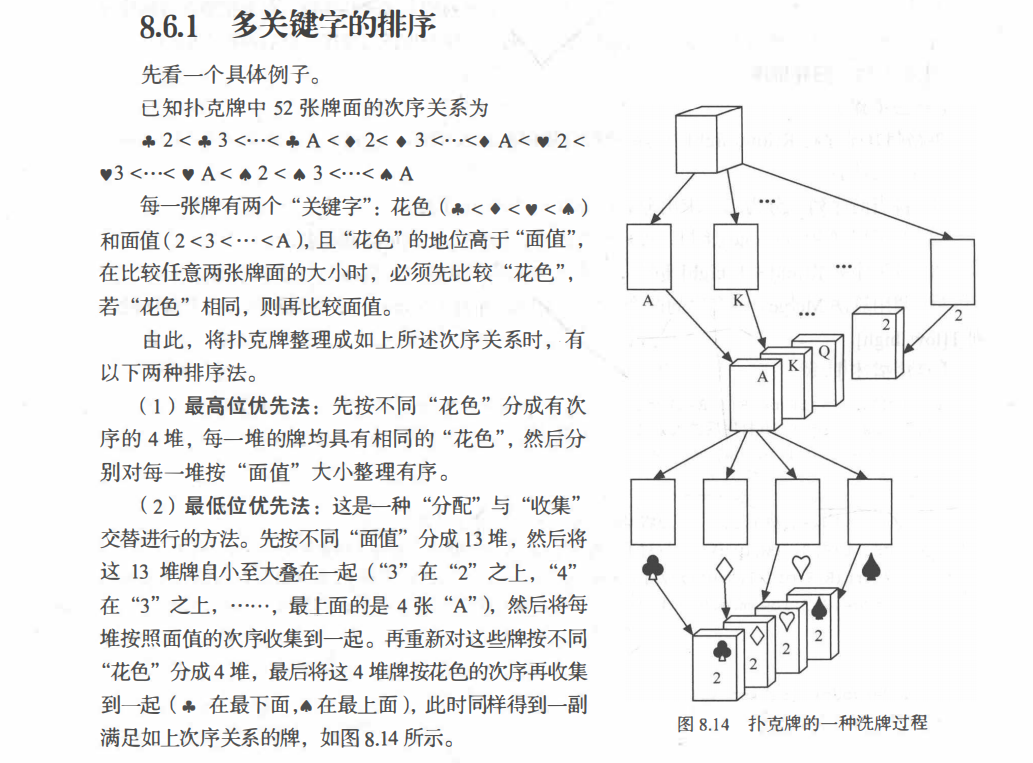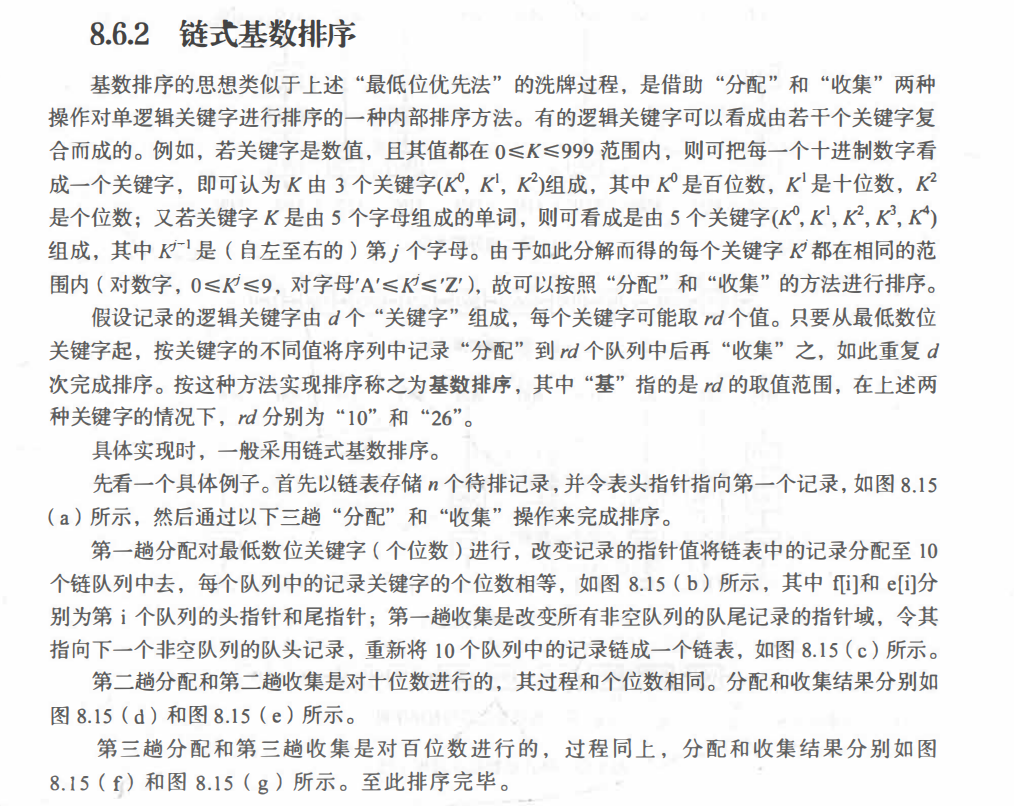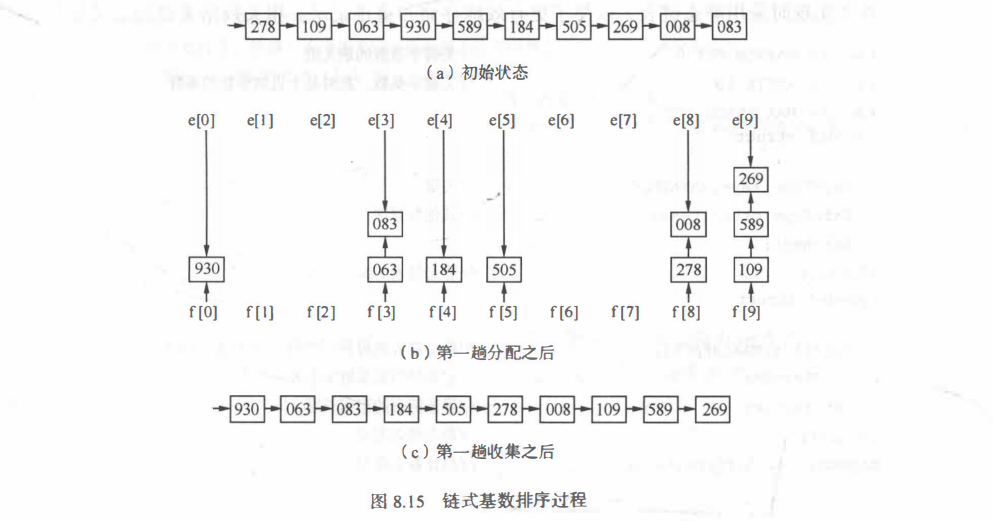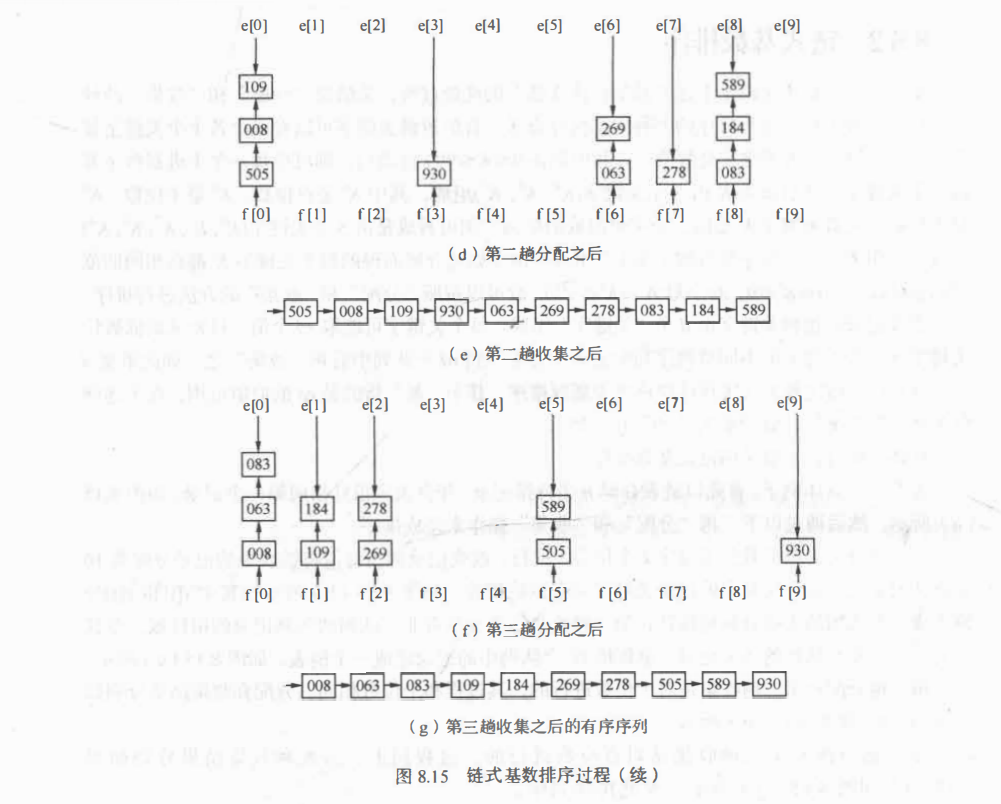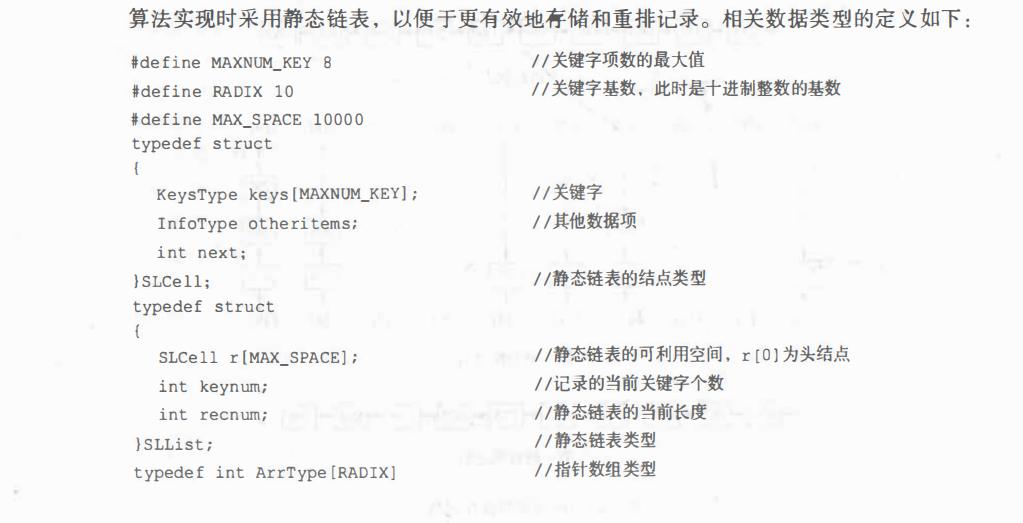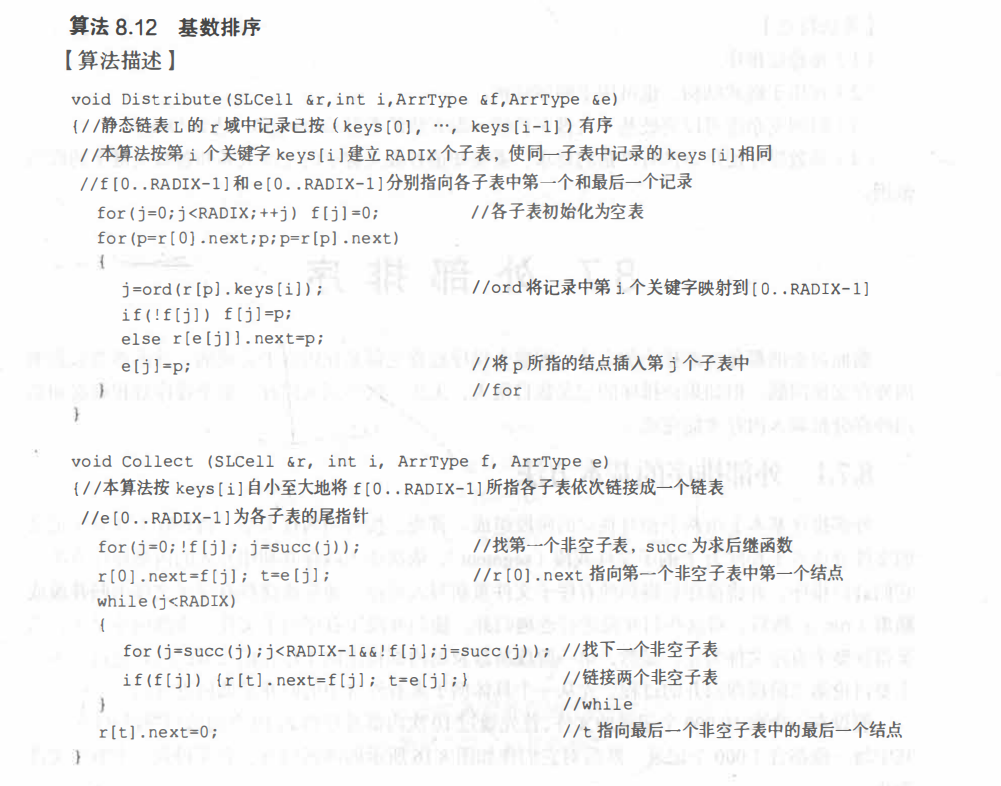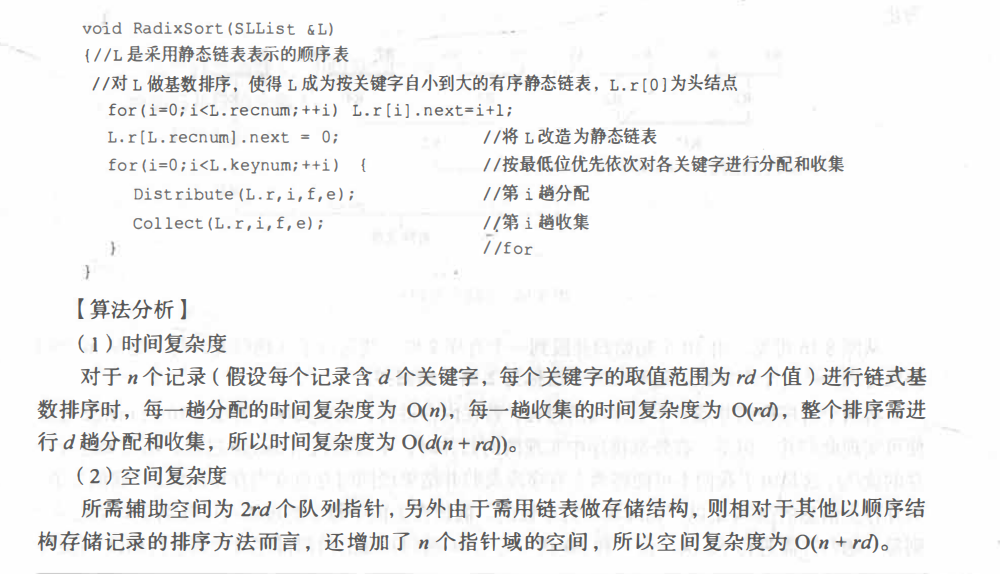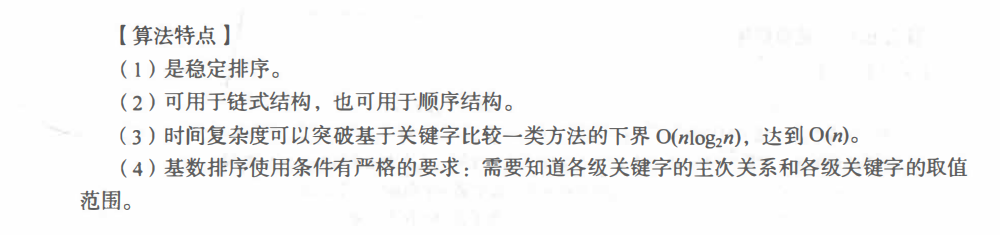*以上内容来自《数据结构（C语言版）（第2版）》人民邮电出版社

展开全文数据结构 排序算法 快速排序
• 排序过程： #include<malloc.h> /* malloc()等 */ #include<stdio.h> /* EOF(=^Z或F6),NULL */ typedef int InfoType; /* 定义其它数据项的类型 */ typedef int KeyType; /* 定义RedType类型的...

# 链式基数排序

### 概述：

基数排序也叫做多关键字排序，基数排序是一种借助“多关键字排序”的思想来实现“单关键字排序”的内部排序算法。

多关键字排序的方法：

n 个记录的序列 {R1, R2, …, Rn} 对关键字 (Ki0, Ki1, …, Ki(d-1) ) 有序是指：对于序列中任意两个记录 Ra 和 Rb  (1≤a < b≤n) 都满足下列（词典）有序关系：(Ka0, Ka1, …, Ka(d-1) ) <  (Kb0, Kb1, …, Kb(d-1) ) ，其中：K0  被称为最主位关键字， K(d-1)  被称为最次位关键字。多关键字排序按照从最主位关键字到最次位关键字或从最次位关键字到最主位关键字的顺序逐次排序，分两种方法：

最高位优先法(Most Significant Digit first)，简称 MSD 法：先按 k0 排序分组，同一组中记录，关键字 k0 相等，再对各组按 k1 排序分成子组，之后，对后面的关键字继续这样的排序分组，直到按最次位关键字  kd  对各子组排序后，再将各组连接起来，便得到一个有序序列。

最低位优先法(Least Significant Digit first)，简称 LSD 法：先从 k(d-1) 开始排序，再对 k(d-2) 进行排序，依次重复，直到对 k0 排序后便得到一个有序序列。

注意一点：LSD的基数排序适用于位数少的数列，如果位数多的话，使用MSD的效率会比较好。

### 排序过程：

注：这里使用LSD法进行排序

例：对于关键字序列 { 278, 109, 063, 930, 589, 184, 505, 269, 008,083 } 进行基数排序 。

可以将每个关键字 K 看成由三个单关键字组成，即 K= k1k2k3， 每个关键字的取值范围为 0≤ki≤9，所以每个关键字可取值的数目为 10。通常将关键字取值的数目称为基数，用 r 表示，在本例中 r =10。

对于关键字序列（AB, BD, ED）可以将每个关键字看成是由二个单字母关键字组成的复合关键字，并且每个关键字的取值范围为 “A~Z”，所以关键字的基数 r = 26。

对于由 d 位关键字组成的复合关键字，需要经过d 趟的“分配”与“收集”。 因此，若 d 值较大，基数排序的时间效率就会随之降低。

在计算机上实现基数排序时，为减少所需辅助存储空间，应采用链表存储 n 个待排记录，即链式基数排序。因为每个桶内的元素个数是未知的，所以需要借助链表结构来实施分配时向桶内仍记录的过程。

在每一趟分配进行时，改变记录的指针值，将链表中的记录分配到 10 个链队列中去，其中 f[i] 和 e[i] 分别为第 i 个队列的头指针和尾指针。具体作法为：

1、以静态链表存储待排记录，并令表头指针指向第一个记录；

2、“分配” 时，按当前“关键字位”所取值，将记录分配到不同的 “链队列” 中，每个队列中记录的 “关键字位” 相同；

3、“收集”时，按当前关键字位取值从小到大将各队列首尾相链成一个链表；

4、对每个关键字位均重复 2 和 3 两步。

• 初始转态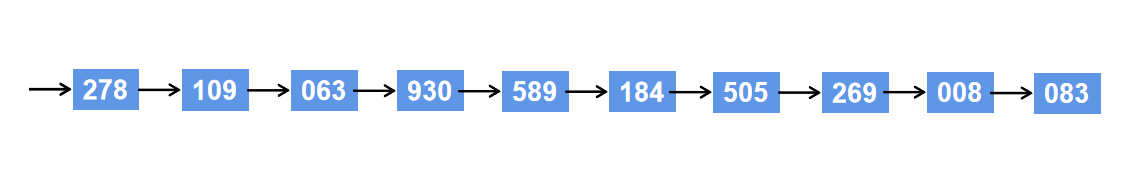• 首先按照各个数据的个位数字分配到0-9的10个区间内，第一趟分配之后结果如下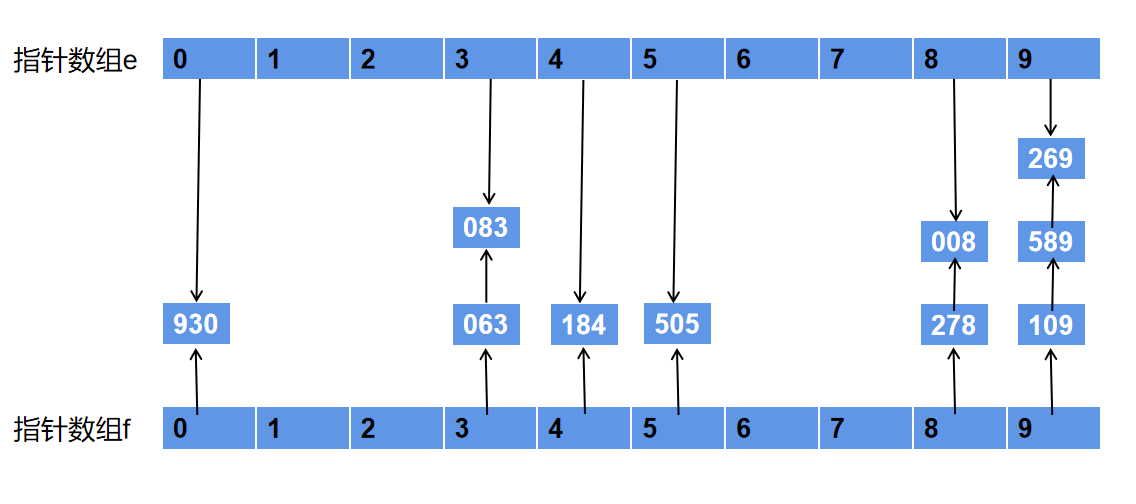• 分配结束后。接下来将所有空间中的数据按照序号由小到大依次重新收集起来，得到如下仍然无序的数据序列：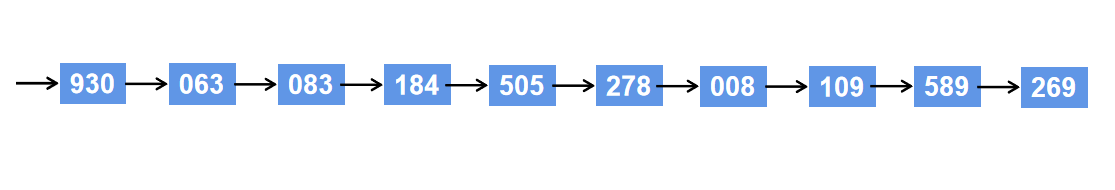• 这次按照十位数字进行分配，步骤同上，第二趟分配之后结果如下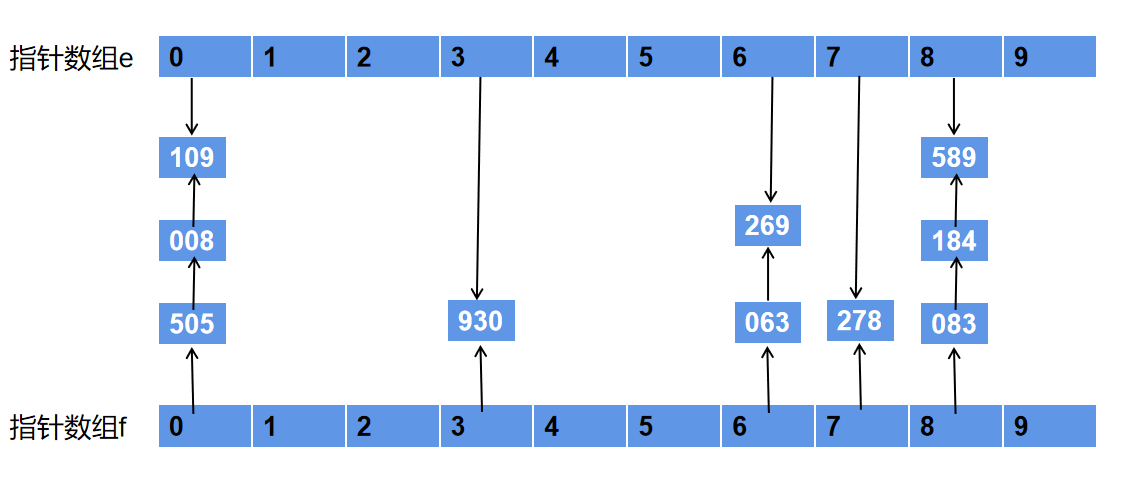• 分配结束后。接下来将所有空间中的数据按照序号由小到大依次再次收集起来，得到如下仍然无序的数据序列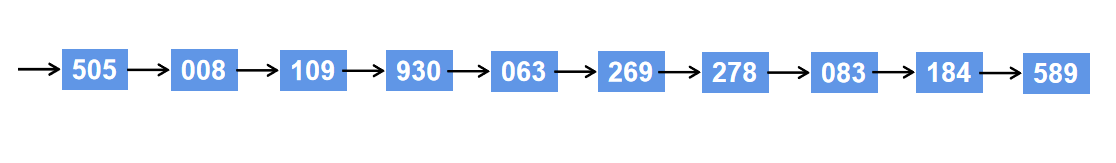• 这次按照百位数字进行分配，步骤同上，第三趟分配之后结果如下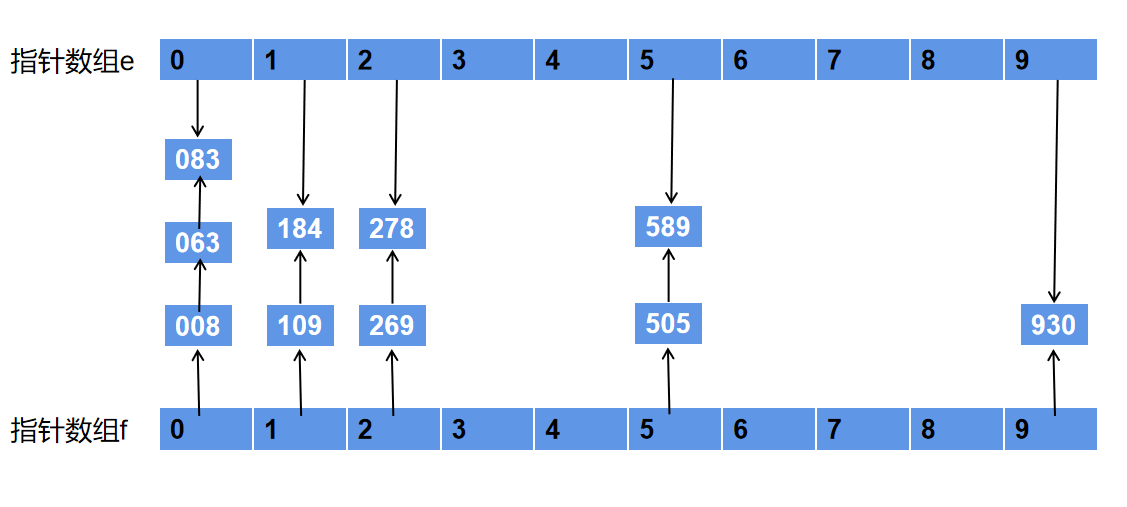• 分配结束后。接下来将所有空间中的数据按照序号由小到大依次再次收集起来，得到有序序列。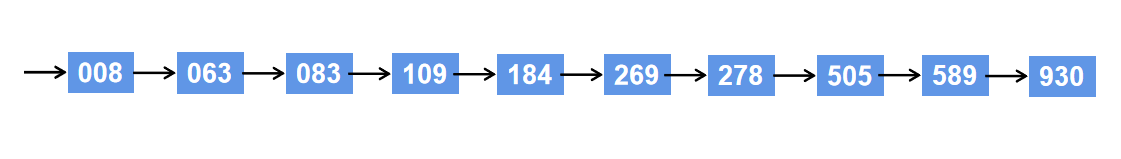### 算法性能：

借助桶编号（键）经过多次分配和采集，最终得到一个有序序列，在这个算法排序过程中，没有经过任何记录的比较，因此基数排序是很独特的排序算法。

时间复杂度：

待排序列为n个记录，d个关键码，关键码的取值范围为 r，其中，一趟分配时间复杂度为 O(n)，一趟收集时间复杂度为O(r)，共进行 d 趟分配和收集，所以链式基数排序的时间复杂度为 O( d·(n+r) ) 。

注意：这不是说这个时间复杂度一定优于O(n·log(n))，因为 d 的大小一般会受到 n 的影响。

空间复杂度：

（2r 个队列指针 + n 个指针域空间），因为一个桶本质是一个链式队列，一共 r 个桶，每个队列有队头和队尾两个指针，就是2r 个队列指针。又原来的待排序列是一个单链表，那么自然需要 n 个next指针控件。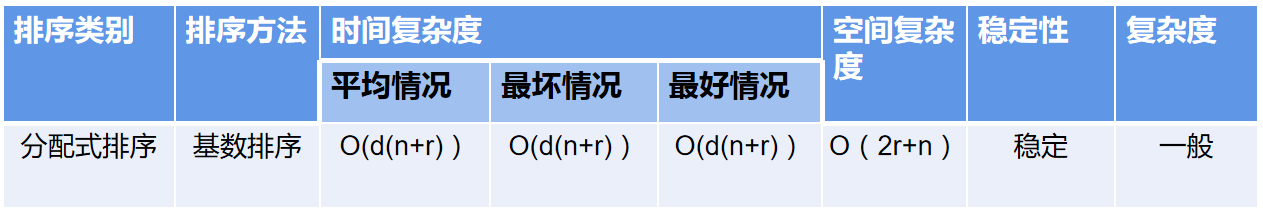### 代码：

#include<malloc.h> /* malloc()等 */
#include<stdio.h> /* EOF(=^Z或F6),NULL */

typedef int InfoType; /* 定义其它数据项的类型 */
typedef int KeyType; /* 定义RedType类型的关键字为整型 */
typedef struct
{
KeyType key; /* 关键字项 */
InfoType otherinfo; /* 其它数据项 */
}RedType; /* 记录类型(同c10-1.h) */
typedef char KeysType; /* 定义关键字类型为字符型 */

/* ---------------------------    基数排序的数据类型     ------------------------------*/

#define MAX_NUM_OF_KEY 8 /* 关键字项数的最大值 */
#define RADIX 10 /* 关键字基数，此时是十进制整数的基数 */
#define MAX_SPACE 1000
typedef struct
{
KeysType keys[MAX_NUM_OF_KEY]; /* 关键字 */
InfoType otheritems; /* 其它数据项 */
int next;
}SLCell; /* 静态链表的结点类型 */

typedef struct
{
SLCell r[MAX_SPACE]; /* 静态链表的可利用空间，r为头结点 */
int keynum; /* 记录的当前关键字个数 */
int recnum; /*  静态链表的当前长度 */
}SLList; /* 静态链表类型 */

typedef int ArrType[RADIX]; /* 指针数组类型 */

/* ------------------------------------------------------------------------------------------*/

void InitList(SLList *L, RedType D[], int n)
{ /* 初始化静态链表L（把数组D中的数据存于L中） */
char c[MAX_NUM_OF_KEY], c1[MAX_NUM_OF_KEY];
int i, j, max = D.key; /* max为关键字的最大值 */
for (i = 1; i < n; i++)
if (max < D[i].key)
max = D[i].key;
(*L).keynum = (int)(ceil(log10(max)));
(*L).recnum = n;
for (i = 1; i <= n; i++)
{
(*L).r[i].otheritems = D[i - 1].otherinfo;
itoa(D[i - 1].key, c, 10); /* 将10进制整型转化为字符型,存入c */
for (j = strlen(c); j < (*L).keynum; j++) /* 若c的长度<max的位数,在c前补'0' */
{
strcpy(c1, "0");
strcat(c1, c);
strcpy(c, c1);
}
for (j = 0; j < (*L).keynum; j++)
(*L).r[i].keys[j] = c[(*L).keynum - 1 - j];
}
}

int ord(char c)
{ /* 返回k的映射(个位整数) */
return c - '0';
}

void Distribute(SLCell r[], int i, ArrType f, ArrType e) /* 算法10.15 */
{ /* 静态键表L的r域中记录已按(keys,...,keys[i-1])有序。本算法按 */
int j, p;
for (j = 0; j < RADIX; ++j)
f[j] = 0; /* 各子表初始化为空表 */
for (p = r.next; p; p = r[p].next)
{
j = ord(r[p].keys[i]); /* ord将记录中第i个关键字映射到[0..RADIX-1] */
if (!f[j])
f[j] = p;
else
r[e[j]].next = p;
e[j] = p; /* 将p所指的结点插入第j个子表中 */
}
}

int succ(int i)
{ /* 求后继函数 */
return ++i;
}

void Collect(SLCell r[], ArrType f, ArrType e)
{ /* 本算法按keys[i]自小至大地将f[0..RADIX-1]所指各子表依次链接成 */
int j, t;
for (j = 0; !f[j]; j = succ(j)); /* 找第一个非空子表，succ为求后继函数 */
r.next = f[j];
t = e[j]; /* r.next指向第一个非空子表中第一个结点 */
while (j < RADIX - 1)
{
for (j = succ(j); j < RADIX - 1 && !f[j]; j = succ(j)); /* 找下一个非空子表 */
if (f[j])
{ /* 链接两个非空子表 */
r[t].next = f[j];
t = e[j];
}
}
r[t].next = 0; /* t指向最后一个非空子表中的最后一个结点 */
}

void printl(SLList L)
{ /* 按链表输出静态链表 */
int i = L.r.next, j;
while (i)
{
for (j = L.keynum - 1; j >= 0; j--)
printf("%c", L.r[i].keys[j]);
printf(" ");
i = L.r[i].next;
}
}

{ /* L是采用静态链表表示的顺序表。对L作基数排序，使得L成为按关键字 */
/* 自小到大的有序静态链表，L.r为头结点。算法10.17 */
int i;
ArrType f, e;
for (i = 0; i < (*L).recnum; ++i)
(*L).r[i].next = i + 1;
(*L).r[(*L).recnum].next = 0; /* 将L改造为静态链表 */
for (i = 0; i < (*L).keynum; ++i)
{ /* 按最低位优先依次对各关键字进行分配和收集 */
Distribute((*L).r, i, f, e); /* 第i趟分配 */
Collect((*L).r, f, e); /* 第i趟收集 */
printf("第%d趟收集后:\n", i + 1);
printl(*L);
printf("\n");
}
}

void print(SLList L)
{ /* 按数组序号输出静态链表 */
int i, j;
printf("keynum=%d recnum=%d\n", L.keynum, L.recnum);
for (i = 1; i <= L.recnum; i++)
{
printf("keys=");
for (j = L.keynum - 1; j >= 0; j--)
printf("%c", L.r[i].keys[j]);
printf(" otheritems=%d next=%d\n", L.r[i].otheritems, L.r[i].next);
}
}

void Sort(SLList L, int adr[]) /* 改此句(类型) */
int i = 1, p = L.r.next;
while (p)
{
p = L.r[p].next;
}
}

void Rearrange(SLList *L, int adr[]) /* 改此句(类型) */
int i, j, k;
for (i = 1; i < (*L).recnum; ++i) /* 改此句(类型) */
if (adr[i] != i)
{
j = i;
(*L).r = (*L).r[i]; /* 暂存记录(*L).r[i] */
while (adr[j] != i)
(*L).r[j] = (*L).r[k];
j = k; /* 记录按序到位 */
}
(*L).r[j] = (*L).r;
}
}

#define N 10
void main()
{
RedType d[N] = { {278,1},{109,2},{63,3},{930,4},{589,5},{184,6},{505,7},{269,8},{8,9},{83,10} };
SLList l;
InitList(&l, d, N);
printf("排序前(next域还没赋值):\n");
print(l);
printf("排序后(静态链表):\n");
print(l);
adr = (int*)malloc((l.recnum) * sizeof(int));
printf("排序后(重排记录):\n");
print(l);
}

运行结果：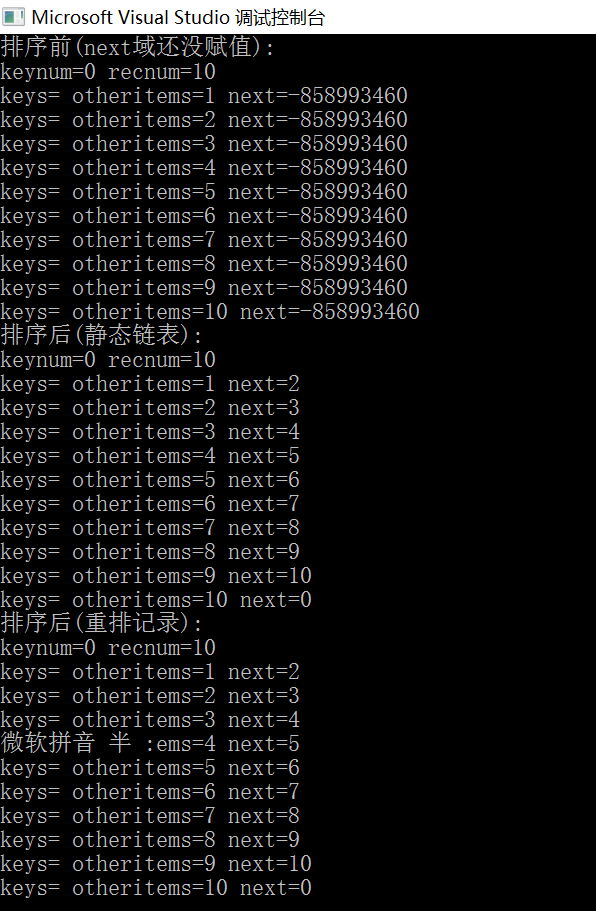展开全文数据结构
• ## 排序-链式基数排序

千次阅读 2018-10-19 09:18:46
基数排序是和前面所述各类排序方法完全不相同的一种排序方法。 从前面几篇文章的讨论可见，实现排序主要是通过关键字之间的比较和移动这两种操作。而实现基数排序不需要进行记录关键字间的比较。基数排序是一种借助...
• ## 链式基数排序

千次阅读 2021-04-22 16:22:02
链式基数排序 基本策略 分配----收集 何为链式基数排序？ 将单关键字排序转成多关建字排序，具体为，在排序过程中，将关键字拆分成若干项，然后把每一项作为一个“关键字“。 对于整数或字符串型的关键字，可将其...指针 排序算法
• 该算法用C++语言实现了基数排序算法，已经调试通过，在Linux系统环境中运行结果正常
• 排序9.6 基数排序9.6.1 多关键字排序9.6.2 链式基数排序 9.6 基数排序 基数排序又被称为桶排序。与前面介绍的几种排序方法相比较，基数排序和它们有明显的不同。前面所介绍的排序方法都是建立在对数据元素关键字进行...数据结构
• 链式基数排序 “分配” 和 “收集” 的实际操作仅为修改链表中的指针和设置队列的头尾指针。 算法如下 #define MAX_NUM_OF_KEY 8 //关键字项数的最大值 #define RADIX 10 // 关键字基数，此时是十进制整数的...
• 文字描述 　基数排序是和前面各类排序方法完全不相同，...先介绍下什么是多关键字排序，以引入链式基数排序算法。 　先介绍什么是多关键字排序： 　比如，对扑克牌进行排序，每张扑克牌有两个“关键字”：花色...
• 【基数排序】 基数排序的算法思想：基数排序不同于前面的各种排序算法，前面的排序算法都是基于元素之间的比较好实现的，而基数排序...因此基数排序算法分成两个分部：分配和收集。 具体算法描述：假设第i个元素a_...基数排序
• 基数排序是八大排序算法之一。 它在棋牌游戏中的应用非常常见。基数排序是采用“分配”与“收集”的办法，用对多关键码进行排序的思想实现对单关键码进行排序的方法。 思路：使用链表存储数据，通过调整指针实现...排序算法
• packagejxau.blueDot.lyx;.../****@authorlyx*@下午2:15:41*@TODO:*基数排序——多关键字排序*多关键字排序的思路是将待排数据里德排序关键字拆分成多个排序关键字；*第1个排序关键字，第2个排序关...
• 基数排序 桶排序 首先来看看桶排序： 为什么桶排序的时间复杂度是O(M+N)？ 因为分配的时间复杂度是M（也就是桶的数量），而收集起来的时间复杂度是N，因此最终时间复杂度是O(M+N)。 上面那种是理想的桶排序，因为...数据结构
• 我不生产代码，我只是代码的搬运工基数排序(radix sort)又称桶排序(bucket sort)，相对于常见的比较排序，基数排序是一种分配式排序，即通过将所有数字分配到应在的位置最后再覆盖到原数组完成排序的过程。...
• # 基本思想基数排序(radix sort)，同样时一种非比较的内部排序算法，主要基于多关键字排序的思想进行排序，它将单个关键字按照基数分成“多个关键字”进行排序。例如整数789是一个关键...数据结构 排序算法
• 链式基数排序 基数排序(Radix Sort)是桶排序的扩展，它的基本思想是：将整数按位数切割成不同的数字，然后按每个位数分别比较。基数排序又称“桶子法”排序，它是根据待排序的每一位上的数字进行入“桶”排序，桶的...基数排序
• 基数排序是借助“分配”和“收集”两种操作进行排序的一种内部排序算法，对已知的数据进行基数排序。 Input 输入数据有多组，对于每组测试数据 第一行一个整数n(n <= 20000)，表示有n个整数要进行排序，第二行n个...数据结构 基数排序
• package cn.itcast.sort; import java.util.ArrayList; import java.util.List; /**  *   * 请对整数序列进行排序。  随机产生1000个整数，其中...排序时使用十个动态数组为临时空间，进行分配和收集。整数 排序 基数
• 实现以下常用的内部排序算法并进行性能比较："直接插入排序"," 折半插入排序"," 2—路插入排序"," 表插入排序"," 希尔排序"," 起泡排序"," 快速排序"," 简单选择排序"," 树形选择排序"," 堆排序"," 归并排序"," 链式...
• 基数排序是采用“分配”与“收集”的办法，用对多关键码进行排序的思想实现对单关键码进行排序的方法。 多关键码排序： 1.以扑克牌排序为例。每张扑克牌有两个“关键码”：花色和面值。其有序关系为： 花色： ...数据结构
• 代码如下: #include <iostream> using namespace std; const int END = -1; const int radix = 10; typedef int KeyType; typedef struct Node { KeyType key; struct Node *next;...JLNode *Creat_RanLin指针
• 文章目录C语言实现基数排序基数排序算法1.定义链结构2.定义链队列结构3.初始化带头结点的链队列4.判断带头结点的链队列是否为空5.带头结点的链队列入队操作6.带头结点的链队列出队操作7.取a的个位、十位、百位........基数排序
• /****************************************************************************************************************... 采用单链表实现的【链式基数排序】 *****************************************************数据结构 排序算法
• 基数排序不同于其他的排序算法，它不是基于比较的算法。基数排序是一种借助多关键字排序的思想对单逻辑关键字进行排序的方法。它是一种稳定的排序算法。多关键字排序中有两种方法：最高位优先法(MSD)和最低位优先法...基数排序 排序算法 桶排序
• 本文内容源于对《数据结构（C语言版）》（第2版）、王道讲解学习所得心得、笔记整理和总结。基数排序：是典型的分配类排序（不同于前面所述...基数排序又分为多关键字的排序、链式基数排序。本文主要针对链式基数排序数据结构 排序算法
• 基数排序是一种非比较型整数排序算法，其原理是将整数按位数切割成不同的数字，然后按每个位数分别比较。由于整数也可以表达字符串（比如名字或日期）和特定格式的浮点数，所以基数排序也不是只能使用于整数。 基数...java 排序算法...